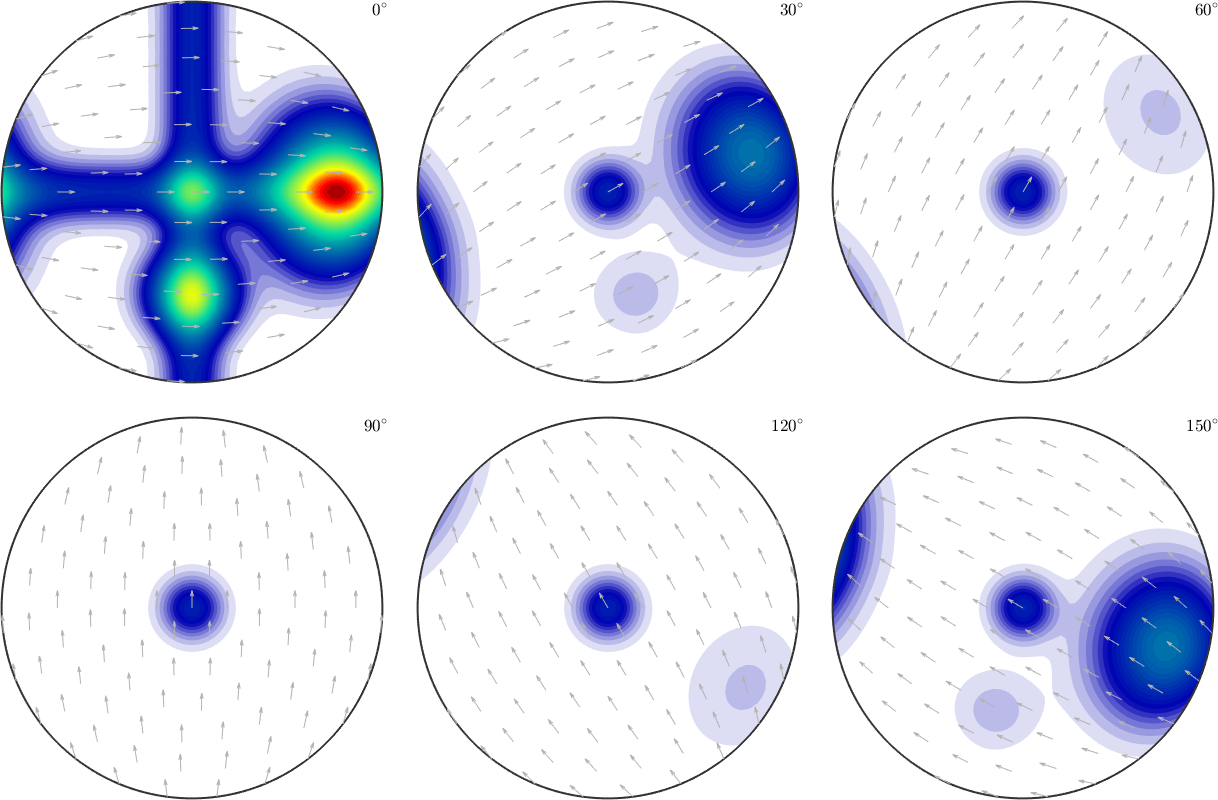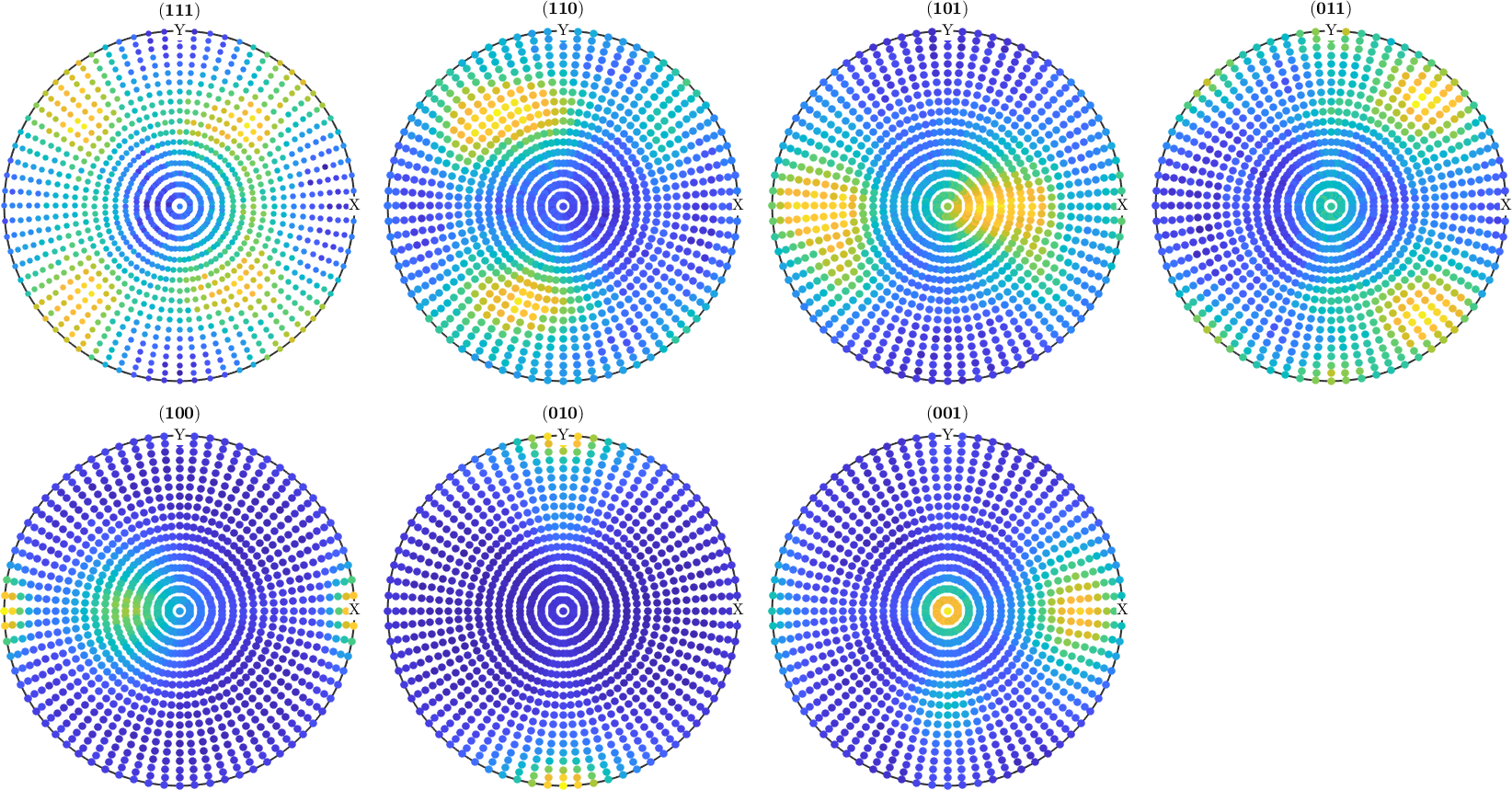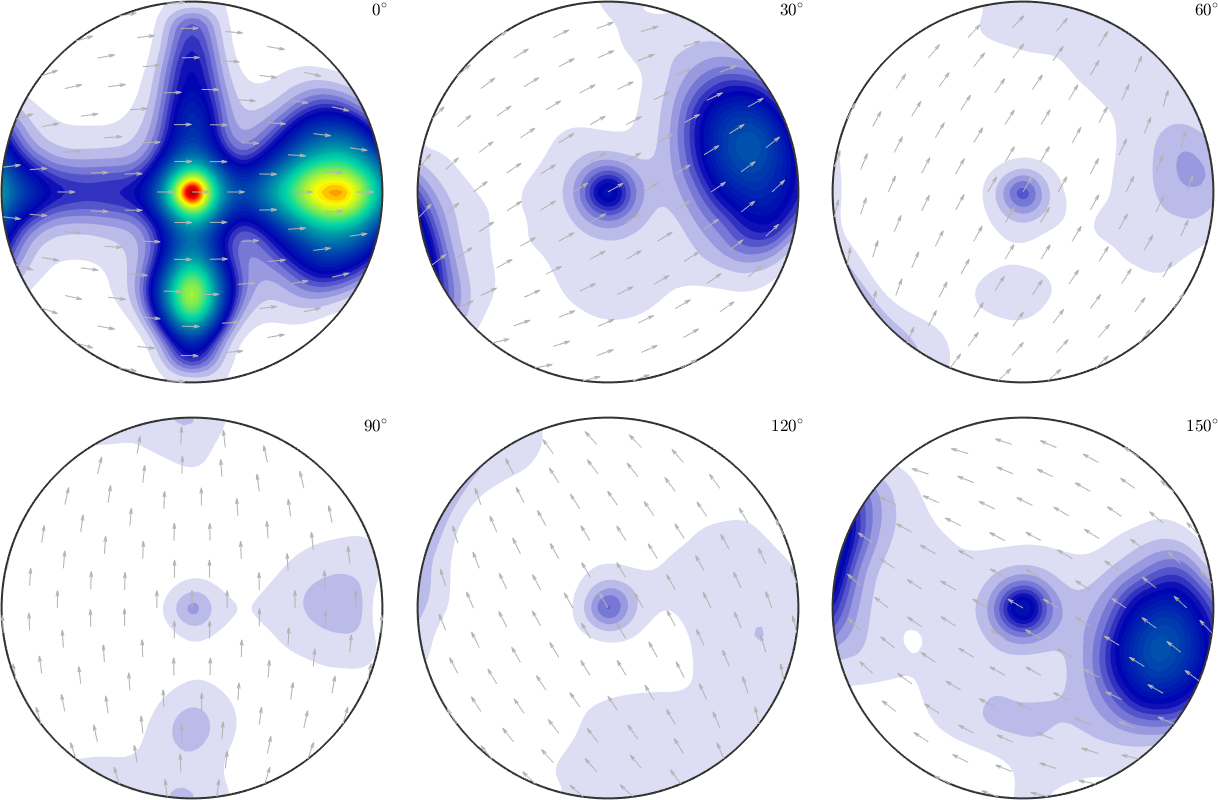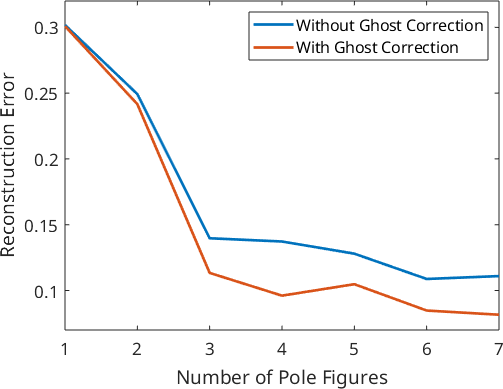Simulating Pole Figure data edit page

Simulating pole figure data from a given ODF is useful to investigate pole figure to ODF reconstruction routines. Let us start with a model ODF given as the superposition of 6 components.In order to simulate pole figure data, the following parameters have to be specified

1. an arbitrary ODF
2. a list of Miller indece
3. a grid of specimen directions
4. superposition coefficients (optional)
5. the magnitude of error (optional)

The list of Miller indece

The grid of specimen directions

Now the pole figures can be simulated using the command calcPoleFigure.

Add some noise to the data. Here we assume that the mean intensity is 1000.

Plot the simulated pole figures.## ODF Estimation from Pole Figure Data

From these simulated pole figures we can now estimate an ODF,

which can be plotted,and compared to the original model ODF.

## Exploration of the relationship between estimation error and number of pole figures

For a more systematic analysis of the estimation error, we vary the number of pole figures used for ODF estimation from 1 to 7 and calculate for any number of pole figures the approximation error. Furthermore, we also apply ghost correction and compare the approximation error to the previous reconstructions.

Plot the error in dependency of the number of single orientations.